# Simple wave modeling and Hilbert Transform in Matlab (codes included)

We demonstrate how to model a simple wave, obtain its frequencies, apply Hilbert transform, and perform edge detection

## Introduction

We can use waves to model almost everything in the world from the thing we can see or touch to the things which we can’t.

Here, we try to model the waves itself.

## Moving Waves

clear; close all; clc
a=1;    %amplitude
f=5;    %frequency
T=1/f;  %time period
w=2*pi*f;   %angular frequency
lb=2*T; %wavelength
k=2*pi/lb; %wavenumber
x=0:pi/200:10*pi;
t=0:0.01:2; %time
figure(1)
for i=1:length(t)
y=a*sin(k*x-w*t(i));    %waveform
plot(x,y)
pause(0.1)
end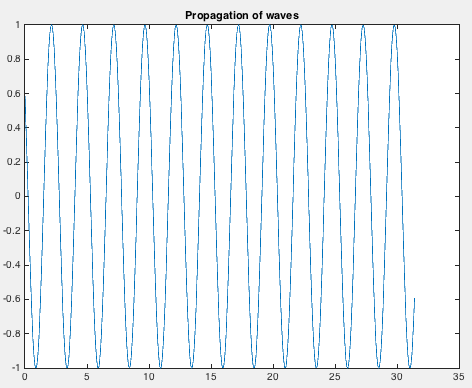## Fourier Transform to analyse the amplitude spectrum

clear; close all; clc
fs=1000;    %sampling frequency
t=0:1/fs:1.5-1/fs;%time
f1=10;  %frequency1
f2=20;  %frequency2
f3=30;  %frequency3
%x=5*sin(2*pi*10*t+3);
x=1*sin(2*pi*f1*t+0.3)+2*sin(2*pi*f2*t+0.2)+3*sin(2*pi*f3*t+0.4);
plot(t,x)
figure(1)
grid on
xlabel('Time')
ylabel('Amplitude')
title('Plot of 2*sin(2*pi*f1*t+0.3)-3*sin(2*pi*f2*t+0.2)+5*cos(2*pi*f3*t+0.4)')
X=fft(x);
fre=fs/length(t);
fre_hz=(0:length(t)/2-1)*fre;
X_mag=abs(X);   %X is complex
figure(2)
plot(fre_hz,X_mag(1:length(t)/2))
grid on
axis([0 40 -inf inf])
xlabel('Frequency (in hz)')
ylabel('Magnitude')
title('Magnitude spectrum of 5*sin(2*pi*10*t+3)')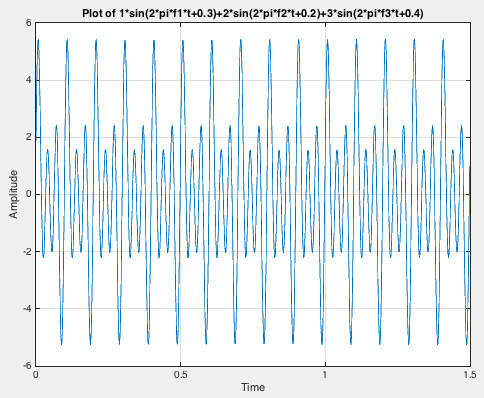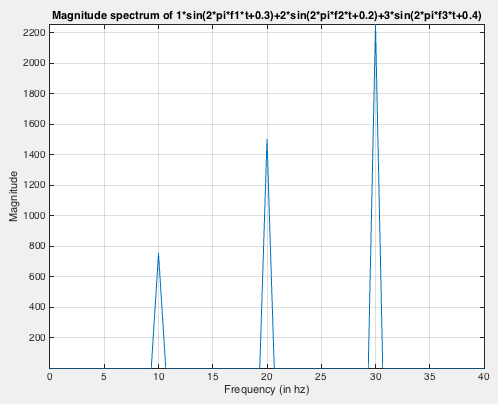## Hilbert Transform and get the envelope of the waveform

clear; close all; clc
fs = 1e4;   %sampling frequency
t = 0:1/fs:1;   %time
f1=10;
f2=20;
f3=30;
x=1*sin(2*pi*f1*t+0.3)+2*sin(2*pi*f2*t+0.2)+3*sin(2*pi*f3*t+0.4);
%x=5*sin(2*pi*10*t+3);
y = hilbert(x);
figure(1)
plot(t,real(y),t,imag(y))
% %xlim([0.01 0.03])
legend('real','imaginary')
title('Hilbert Function')
figure(2)
env=abs(y);
plot(t,x)
xlabel('Time')
title('Envelope')
hold on
plot(t,env)
legend('original','envelope')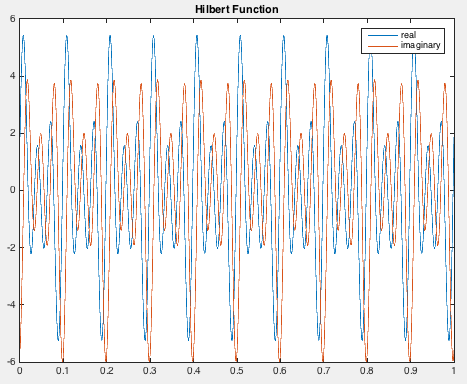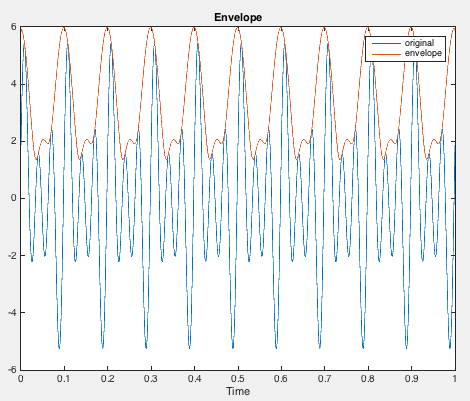## Application of Hilbert Transform: Edge Detection and comparison with the classical derivative method

clear; close all; clc
x = 0:0.1:100;
y = channel(x,[10 30 70]);  %channel function to define a trapezoidal channel
plot(x,y)
figure(1)
subplot(311)
plot(x,y)
grid on
title('Channel')

subplot(312)
deriv =diff(y); %dervative of the channel
plot(x(2:end),deriv)
title('Detection by derivative method')
grid on

subplot(313)
hil = hilbert(y);   %hilbert transform of the channel
env=abs(hil);
plot(x,env)
grid on
title('Detection by hilbert transform')

• Channel Function
function y = channel(x, params)

a = params(1); b = params(2); c = params(3);
for index=1:length(x)
if x(index)<=a
y(index)=0;
elseif (x(index) >= a) && (x(index) <= b)
y(index)=(x(index)-a)/(a-b);
elseif (x(index) >= b) && (x(index) < c)
y(index)=-1;
elseif (x(index) >= c)
y(index)=0;
end
end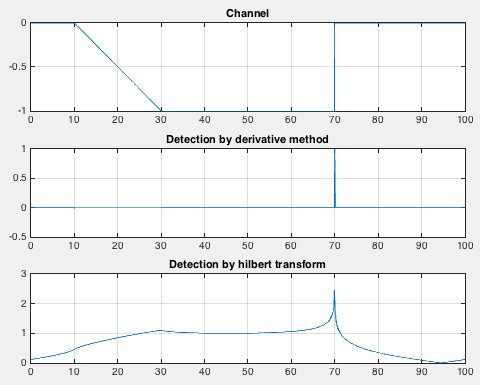## Complex Moving Waves

In nature, usually we encounter waves as an ensemble of many frequencies.

Here, let us try to add more frequencies in the previous scenario. We plot a wave containing three frequencies.

clear; close all; clc
fs=1000;    %sampling frequency
t=0:1/fs:0.5-1/fs;%time
f=[1 2 3];  %frequency1
a=[1 2 3];  %amplitude
c=2; %wave speed
T=1./f;  %time period
w=2*pi*f;   %angular frequency
lb=c*T; %wavelength
k=(2*pi)./lb; %wavenumber
x=0:pi/200:(2.5*pi)-pi/200;
figure('Position',[440 378 800 500])
for i=1:length(t)/2
%     y=a*sin(k*x-w*t(i));    %waveform
y=a(1)*sin((k(1)*x)-(w(1)*t(i))+0.3)+a(2)*sin((k(2)*x)-(w(2)*t(i))+0.4)+a(3)*sin((k(3)*x)-(w(3)*t(i))+0.5);
plot(x,y,'--*')
title('Propagation of waves')
xlabel('x')
ylabel('Amplitude')
grid on
pause(0.05)
endWe have modelled the wave in which each frequency is travelling with the same velocity. We can add some complexity where every frequency in our wave is travelling with different speed. This is popularly known as dispersion.

Let us model our waves such that the wave speed for 1,2 and 3 Hz is 0.5,1 and 2 km/s.In this case, we can notice that the waves are travelling in groups and its shape keep changing. We can use the concept of hilbert transform to model the propagation of the group.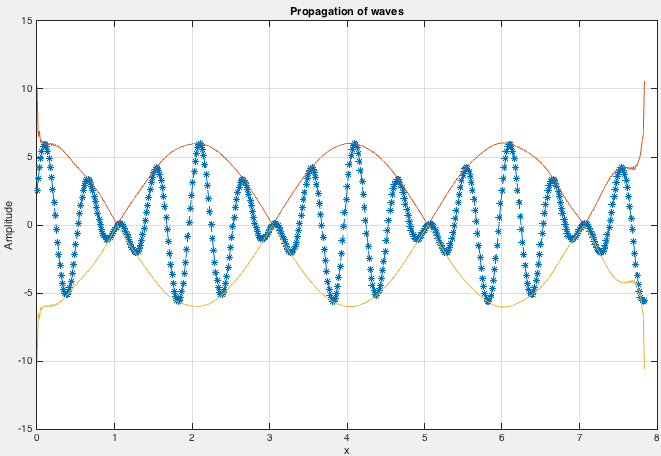Tags:

Categories:

Created on:#### Disclaimer of liability

The information provided by the Earth Inversion is made available for educational purposes only.

Whilst we endeavor to keep the information up-to-date and correct. Earth Inversion makes no representations or warranties of any kind, express or implied about the completeness, accuracy, reliability, suitability or availability with respect to the website or the information, products, services or related graphics content on the website for any purpose.

UNDER NO CIRCUMSTANCE SHALL WE HAVE ANY LIABILITY TO YOU FOR ANY LOSS OR DAMAGE OF ANY KIND INCURRED AS A RESULT OF THE USE OF THE SITE OR RELIANCE ON ANY INFORMATION PROVIDED ON THE SITE. ANY RELIANCE YOU PLACED ON SUCH MATERIAL IS THEREFORE STRICTLY AT YOUR OWN RISK.